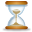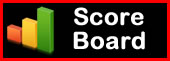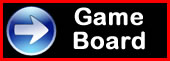SuperTeacherTools# Join Code:UF77C6

How to Play:

Instant Jeopardy Review is designed for live play with as many individuals or teams as you like! Each team will need to enter the Join Code above. Teams choose a question, then try to give the best answer.

Scoring is built in for each team.

You can also choose to use a timer below.

Have fun!

Play This Game Live NowSet to X to hide the timer

Prefer the old Flash template? Switch now: Arithmetic and Geometric Sequences Jeopardy Review Flash Version

Arithmetic and Geometric Sequences

 Common Difference/Ratio First Five Terms from Recursive Formula Give the Recursive Formula Give the Explicit Formula Find the 30th term 10 10 10 10 10 20 20 20 20 20 30 30 30 30 30 40 40 40 40 40 50 50 50 50 50 Final Questiond=7
d=-11
r=3
r=-4
r=1/3
7, 13, 19, 25, 31
30, 21, 12, 3, -6
-4, -16, -64, -256, -1024
1, -8, 64, -512, 4096
96, 48, 24, 12, 6
a(1)=-4 a(n)=a(n-1)+2
a(1)=10 a(n)=a(n-1)-3
a(1)=3 a(n)=2a(n-1)
a(1)=-2 a(n)=-5a(n-1)
a(1)=80 a(n)=1/2a(n-1)
a(n)=-7+(n-1)(-3)
a(n)=5+(n-1)(-4)
a(n)=19+(n-1)(9)
a(n)=-6+(n-1)(5)
a(n)=8+(n-1)(-6)
80
-121
280
139
-166Remove Teams / Players